## PRogramming

 Question 1
Consider the following C program:
#include <stdio.h>
int counter = 0;
int calc (int a, int b) {
int c;
counter++;
if (b==3) return (a*a*a) ;
else {
c = calc (a, b/3) ;
return (c*c*c) ;
}
}
int main () {
calc (4, 81);
printf ("%d", counter) ;
}
The output of this program is ______.
 A 4 B 5 C 6 D 7
Programming       Programming       GATE 2018       Video-Explanation
Question 1 Explanation:Question 2

Consider the following C program.

```#include <stdio.h>
struct Outnode {
char x, y, z ;
};

int main () {
struct Outnode p = {'1', '0', 'a'+2} ;
struct Outnode *q = &p ;
printf ("%c, %c", *((char*)q+1), *((char*)q+2)) ;
return 0 ;
}
```

The output of this program is

 A 0, c B 0, a+2 C ‘0’, ‘a+2’ D ‘0’, ‘c’
Programming       Programming       GATE 2018       Video-Explanation
Question 2 Explanation:
char x = ‘a’+2;
The x variable here stores a character ‘c’ in it.
Because +2 will increment ascii value of a from 92 to 95.
Hence the structure p contains 3 character values and they are ‘1’, ‘0’, and ‘c’.
q is a pointer pointing to structure p.
Hence q is pointing to ‘1’, q+1 pointing to ‘0’ and q+2 pointing to ‘c’.
Option d cannot be correct, as though they are characters, printf will not print them in single quotes.
 Question 3

Consider the following C program:

```#include<stdio.h>

void fun1(char *s1, char *s2)  {
char *tmp;
tmp = s1;
s1 = s2;
s2 = tmp;
}

void fun2(char **s2, char **s2)  {
char *tmp;
tmp = *s1;
*s1 = *s2;
*s2 = tmp;
}

int main ()  {
char *str1 = "Hi", *str2 = "Bye";
fun1(str1, str2);     printf("%s %s", str1, str2);
fun2(&str1, &str2);   printf("%s %s", str1, str2);
return 0;
}
```

The output of the program above is

 A Hi Bye Bye Hi B Hi Bye Hi Bye C Bye Hi Hi Bye D Bye Hi Bye Hi
Programming       Programming       GATE 2018       Video-Explanation
Question 3 Explanation:
The first call to the function ‘func1(str1, str2);’ is call by value.
Hence, any change in the formal parameters are NOT reflected in actual parameters.
Hence, str1 points at “hi” and str2 points at “bye”.
The second call to the function ‘func2(&str1, &str2);’ is call by reference.
Hence, any change in formal parameters are reflected in actual parameters.
Hence, str1 now points at “bye” and str2 points at “hi”.
Hence answer is “hi bye bye hi”.
 Question 4

Consider the following C code. Assume that unsigned long int type length is 64 bits.

```        unsigned long int fun(unsigned long int n)  {
unsigned long int i, j, j=0, sum = 0;
for (i = n; i > 1; i = i/2) j++;
for ( ; j > 1; j = j/2) sum++;
return sum;
}
```

The value returned when we call fun with the input 240 is

 A 4 B 5 C 6 D 40
Programming       Programming       GATE 2018       Video-Explanation
Question 4 Explanation:
Since 240 is the input, so first loop will make j = 40.
Next for loop will divide j value (which is 40) by 2, each time until j>1.
j loop starts:
j=40 & sum=1
j=20 & sum=2
j=10 & sum=3
j=5 & sum=4
j=2 & sum=5
j=1 & break
So, sum = 5.
 Question 5

Consider the following program written in pseudo-code. Assume that x and y are integers.

```Count (x, y)  {
if (y != 1)  {
if (x != 1)  {
print("*") ;
Count (x/2, y) ;
}
else  {
y = y - 1 ;
Count (1024, y) ;
}
}
}
```

The number of times that the print statement is executed by the call Count(1024, 1024) is ______.

 A 10230 B 10231 C 10232 D 10233
Programming       PRogramming       GATE 2018       Video-Explanation
Question 5 Explanation:
#include
int count=0;
Count(x,y){
if(y!=1){
if(x!=1){
printf("*");
count = count +1;
Count(x/2,y);
}
else{
y=y-1;
Count(1024,y);
}
}
}
void main()
{
Count(1024,1024);
printf("\n%d\n",count);
}Count ( ) is called recursively for every (y = 1023) & for every y, Count ( ) is called (x = 10) times = 1023 × 10 = 10230
 Question 6

Consider the following C code:

```             #include <stdio.h>
int *assignval(int *x, int val)   {
*x = val;
return x;
}

void main ( )  {
int  *x = malloc(sizeof(int));
if(NULL == x)  return;
x = assignval(x, 0);
if(x)  {
x = (int *)malloc(size of(int));
if(NULL == x)  return;
x = assignval(x, 10);
}
printf("%dn",  *x);
free(x);
}
```

The code suffers from which one of the following problems:

 A compiler error as the return of malloc is not typecast approximately B compiler error because the comparison should be made as x==NULL and not as shown C compiles successfully but execution may result in dangling pointer D compiles successfully but execution may result in memory leak
Programming       Programming       GATE 2017 [Set-1]       Video-Explanation
Question 6 Explanation:
Option A:
In C++, we need to perform type casting, but in C Implicit type casting is done automatically, so there is no compile time error, it prints10 as output.
Option B:
NULL means address 0, if (a == 0) or (0 == a) no problem, though we can neglect this, as it prints 10.
Option C:
x points to a valid memory location. Dangling Pointer means if it points to a memory location which is freed/ deleted.
int*ptr = (int*)malloc(sizeof(int));
free(ptr); //ptr becomes a dangling pointer
ptr = NULL; //Removing Dangling pointers condition
Option D:
x is assigned to some memory location
int*x = malloc(sizeof(int));
→ (int*)malloc(sizeof(int)) again assigns some other location to x, previous memory location is lost because no new reference to that location, resulting in Memory Leak.
Hence, Option D.
 Question 7

Consider the following two functions.

```void fun1(int n)   {                 void fun2(int n)   {
if(n == 0)  return;                  if(n == 0)  return;
printf("%d", n);                     printf("%d", n);
fun2(n - 2);                         fun1(++n);
printf("%d", n);                     printf("%d", n);
}                                    }
```

The output printed when fun1(5) is called is

 A 53423122233445 B 53423120112233 C 53423122132435 D 53423120213243
Programming       Programming       GATE 2017 [Set-1]       Video-Explanation
Question 7 Explanation: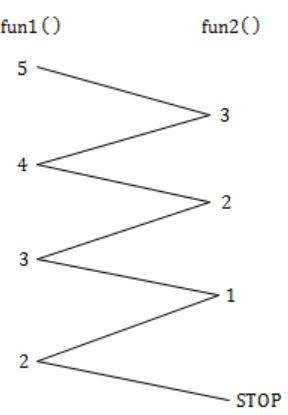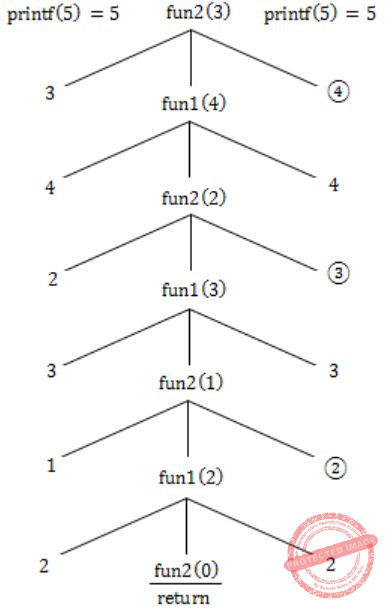In fun2, we increment (pre) the value of n, but in fun1, we are not modifying the value.
Hence increment in value in recursion (back).
Hence, 5 3 4 2 3 1 2 2 2 3 3 4 4 5.
 Question 8

Consider the C functions foo and bar given below:

```         int foo(int val)   {
int x = 0;
while (val>0)   {
x = x + foo(val--);
}
return val;
}

int bar(int val)   {
int x = 0;
while (val>0)   {
x = x + bar(val-1);
}
return val;
}```

Invocations of foo(3) and bar(3) will result in:

 A Return of 6 and 6 respectively. B Infinite loop and abnormal termination respectively. C Abnormal termination and infinite loop respectively. D Both terminating abnormally.
Programming       Programming       GATE 2017 [Set-1]       Video-Explanation
Question 8 Explanation:
while(val>0)
{
x = x + foo(val--);
}
In this case foo(val--) is same as foo(val) & val-- ;
Because the recursive function call is made without changing the passing argument and there is no Base condition which can stop it.
It goes on calling with the same value ‘val’ & the system will run out of memory and hits the segmentation fault or will be terminated abnormally.
The loop will not make any difference here.
while(val>0)
{
x = x + bar(val-1);
}
bar(3) calls bar(2)
bar(2) calls bar(1)
bar(1) calls bar(0) ⇾ Here bar(0) will return 0.
bar(1) calls bar(0)
bar(1) calls bar(0)……..
This will continue.
Here is a problem of infinite loop but not abrupt termination.
Some compilers will forcefully preempt the execution.
 Question 9

Consider the following C program.

```#include <stdio.h>
#include <string.h>

void printlength (char*s, char*t)   {
unsigned int c = 0;
int len = ((strlen(s) - strlen(t)) > c) ? strlen(s):strlen(t);
printf("%d\n", len);
}

void main ()  {
char*x = "abc";
char*y = "defgh";
printlength(x,y);
}
```

Recall that strlen is defined in string.h as returning a value of type size_t, which is an unsigned int. The output of the program is _________.

 A 3 B 4 C 5 D 6
Programming       Programming       GATE 2017 [Set-1]       Video-Explanation
Question 9 Explanation:
main ( )
{
char*x = "abc";
char*y = "defgh";
printlength(x,y);
}
printlength(char*3, char*t)
{
unsigned int c = 0;
int len = ((strlen(s) - strlen(t))> c) ? strlen(s) : strlen(t);
printf("%d", len);
}
Here strlen(s) - strlen(t) = 3 - 5 = -2
But in C programming, when we do operations with two unsigned integers, result is also unsigned. (strlen returns size_t which is unsigned in most of the systems).
So this result '-2' is treated as unsigned and its value is INT_MAX-2.
Now the comparison is in between large number & another unsigned number c, which is 0.
So the comparison returns TRUE here.
Hence (strlen(s) - strlen(t))>0 will return TRUE, on executing, the conditional operator will return strlen(s)
⇒ strlen(abc) = 3
 Question 10

The output of executing the following C program is __________.

```           #include<stdio.h>
int total (int v)   {
static int count=0;
while(v)   {
count += v&1;
v ≫= 1;
}
return count;
}

void main()    {
static int x = 0;
int i = 5;
for(; 1> 0; i--)    {
x = x + total(i);
}
printf("%d\n", x);
}
```
 A 23 B 24 C 25 D 26
Programming       Programming       GATE 2017 [Set-1]       Video-Explanation
Question 10 Explanation:
Arithmetic operators have least priority in this case, as count+=v & 1, we first compute v& 1 and then adds to count variable.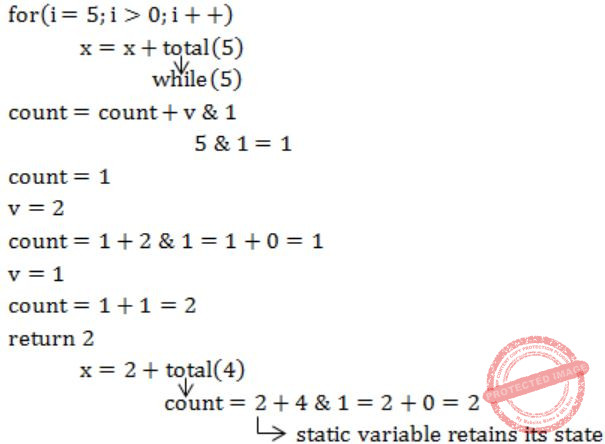Question 11

Consider the following function implemented in C:

```void printxy (int x, int y)   {
int *ptr;
x = 0;
ptr = &x;
y = *ptr;
*ptr = 1;
printf("%d,%d",x,y);
}
```

The output of invoking printxy(1, 1) is

 A 0, 0 B 0, 1 C 1, 0 D 1, 1
Programming       Programming       GATE 2017 [Set-2]       Video-Explanation
Question 11 Explanation:
printxy (int x, int y)
{
int *ptr;
x = 0;
ptr = &x;
y = *ptr;
*ptr = 1;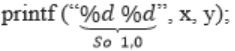}
printxy (1, 1)Question 12

Consider the C program fragment below which is meant to divide x and y using repeated subtractions. The variables x, y, q and r are all unsigned int.

```          while (r >= y)  {
r = r - y;
q = q + 1;
}
```

Which of the following conditions on the variables x, y, q and r before the execution of the fragment will ensure that the loop terminates in a state satisfying the condition x == (y*q + r)?

 A (q == r) && (r == 0) B (x > 0) && (r == x) && (y > 0) C (q == 0) && (r == x) && (y > 0) D (q == 0) && (y > 0)
Programming       Programming       GATE 2017 [Set-2]       Video-Explanation
Question 12 Explanation:
Divide x by y.
x, y, q, r are unsigned integers.
while (r >= y)
{
r = r – y;
q = q + 1;
}
Loop terminates in a state satisfying the condition
x == (y * q + r)
y ⇒ Dividend = Divisor * Quotient + Remainder
So, to divide a number with repeated subtractions, the Quotient should be initialized to 0 and it must be incremented for every subtraction.
So initially q=0 which represents
x = 0 + r ⇒ x = r
and y must be a positive value (>0).
 Question 13

Consider the following snippet of a C program. Assume that swap(&x, &y)  exchanges the contents of x and y.

```int main ()  {
int array[] = {3, 5, 1, 4, 6, 2};
int done = 0;
int i;

while (done == 0)  {
done = 1;
for (i=0; i<=4; i++)  {
if (array[i] < array[i+1])  {
swap (&array[i], &array[i+1]);
done = 0;
}
}
for (i=5; i>=1; i--)  {
if (array[i] > array[i-1])   {
swap(&array[i], &array[i-1]);
done=0;
}
}
}
printf("%d", array);
}
```

The output of the program is ___________.

 A 3 B 4 C 5 D 6
Programming       Programming       GATE 2017 [Set-2]       Video-Explanation
Question 13 Explanation:
Swap (&x, &y) exchanges the contents of x & y.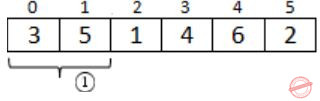(1) ⇒ 1st for i = 0 <= 4
a < a ≃ 3<5 so perform swapping
done =(1) ⇒ no swap (3, 1)
(2) ⇾ perform swap (1, 4)(1) ⇒ perform swap (1, 6)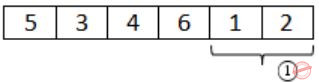(1) ⇒ perform swap (1, 2)(1) ⇒ (done is still 0)
for i = 5 >= 1 a > a ≃ 1>2 – false. So, no swapping to be done
(2) ⇾ no swap (6, 2)
(3) ⇾ swap (4, 6)(1) ⇒ Swap (3, 6)(1) ⇒ Swap (5, 6)⇒ Done is still 0. So while loop executes again.
(1) ⇾ no swap (6, 5)
done = 1
(2) ⇾ No swap (5, 3)
(3) ⇾Swap (3, 4)So, array  = 3
 Question 14

Consider the following C program.

```#include<stdio.h>
#include<string.h>
int main ()  {
char* c = "GATECSIT2017";
char* p = c;
printf("%d", (int) strlen (c + 2[p] - 6[p] - 1));
return 0;
}
```

The output of the program is __________.

 A 1 B 2 C 4 D 6
Programming       Programming       GATE 2017 [Set-2]       Video-Explanation
Question 14 Explanation:
char * C = “GATECSIT2017”;
char * P = C;
(int) strlen (C + 2[P] – 6[P] – 1)C + 2[P] - 6[P] – 1
∵2[P] ≃ P
100 + P – P – 1
100 + T – I – 1
100 + 84 – 73 – 1
ASCII values: T – 84, I – 73
100 + 11 – 1
= 110
(int) strlen (110)
strlen (17) ≃ 2
 Question 15

Consider the following C program.

```void f(int, short);
void main ()
{
int i = 100;
short s = 12;
short *p = &s;
__________ ;         // call to f()
}
```

Which one of the following expressions, when placed in the blank above, will NOT result in a type checking error?

 A f(s, *s) B i = f(i, s) C f(i, *s) D f(i, *p)
Programming       Programming       GATE 2016 [Set-1]       Video-Explanation
Question 15 Explanation:
int i = 100;
short s = 12;
short *p = &s;
_______ // call to f ( ) :: (void f(int,short);)
It is clearly mentioned the return type of f is void.
By doing option elimination
(A) & (C) can be eliminated as s is short variable and not a pointer variable.
(B) i = f(i, s) is false because f’s return type is void, but here shown as int.
(D) f(i, *p)
i = 100
*p = 12
Hence TRUEQuestion 16

Consider the following C program.

```#include<stdio.h>
void mystery(int *ptra, int *ptrb) {
int *temp;
temp = ptrb;
ptrb = ptra;
ptra = temp;
}
int main() {
int a=2016, b=0, c=4, d=42;
mystery(&a, &b);
if (a < c)
mystery(&c, &a);
mystery(&a, &d);
printf("%d\n", a);
}
```

The output of the program is ________.

 A 2016 B 2017 C 2018 D 2019
Programming       Programming       GATE 2016 [Set-1]       Video-Explanation
Question 16 Explanation:For the first mystery (&a, &b);temp = ptr b
ptr b = ptr a
ptr a = temp
If (a The function mystery (int *ptra, int *ptrb) does not change the value of variables pointed by pointers, instead it only changes the addresses inside the pointers, which is in no way going to modify the values of a, b, c, d.
Hence, a = 2016 will be printed.
 Question 17

The following function computes the maximum value contained in an integer array p[] of size n (n >= 1).

```       int max(int *p, int n) {
int a=0, b=n-1;

while (__________) {
if (p[a] <= p[b]) {a = a+1;}
else                 {b = b-1;}
}

return p[a];
}
```

The missing loop condition is

 A a != n B b != 0 C b > (a + 1) D b != a
Programming       Programming       GATE 2016 [Set-1]       Video-Explanation
Question 17 Explanation:
main ( )
{
int arr [ ] = {3, 2, 1, 5, 4};
int n = sizeof(arr) / sizeof (arr);
printf (max(arr, 5));
}
int max (int *p, int n)
{
int a = 0, b = n – 1;
(while (a!=b))
{
if (p[a] <= p[b])
{
a = a + 1;
}
else
{
b =b – 1;
}
}
return p[a];
}
The function computes the maximum value contained in an integer array p[ ] of size n (n >= 1).
If a = = b, means both are at same location & comparison ends.
 Question 18

What will be the output of the following C program?

```void count(int n)  {
static int d=1;

printf("%d ", n);
printf("%d ", d);
d++;
if(n>1) count(n-1);
printf("%d ", d);
}

void main() {
count(3);
}
```
 A 3 1 2 2 1 3 4 4 4 B 3 1 2 1 1 1 2 2 2 C 3 1 2 2 1 3 4 D 3 1 2 1 1 1 2
Programming       Programming       GATE 2016 [Set-1]       Video-Explanation
Question 18 Explanation:Count (3)
static int d = 1
It prints 3, 1
d++; //d = 2
n>1, count(2)
prints 2, 2
d++; // d = 3
n>1, count(1)
prints 1, 3 → Here n = 1, so condition failed & printf (last statement) executes thrice & prints d
d++; //d=4 value as 4. For three function calls, static value retains.
∴ 312213444
 Question 19

What will be the output of the following pseudo-code when parameters are passed by reference and dynamic scoping is assumed?

```       a=3;
void n(x) {x = x * a; print(x);}
void m(y) {a = 1; a = y - a; n(a); print(a);}
void main() {m(a);}
```
 A 6, 2 B 6, 6 C 4, 2 D 4, 4
Programming       Programming       GATE 2016 [Set-1]       Video-Explanation
Question 19 Explanation:First m(a) is implemented, as there are no local variables in main ( ), it takes global a = 3;
m(3) is passed to m(y).
a = 1
a = 3 – 1 = 2
n(2) is passed to n(x).
Since it is dynamic scoping
x = 2 * 2 = 4 (a takes the value of its calling function not the global one).
The local x is now replaced in m(y) also.
Hence, it prints 4,4.
And we know it prints 6, 2 if static scoping is used.
It is by default in C programming.
 Question 20

The value printed by the following program is __________.

```     void f(int* p, int m) {
m = m + 5;
*p = *p + m;
return;
}

void main() {
int i=5, j=10;
f(&i, j);
printf("%d", i+j);
}
```
 A 30 B 31 C 32 D 33
Programming       Programming       GATE 2016 [Set-2]       Video-Explanation
Question 20 Explanation:P is a pointer stores the address of i, & m is the formal parameter of j.
Now, m = m + 5;
*p = *p + m;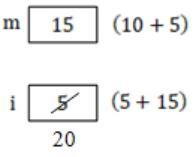Hence, i + j will be 20 + 10 = 30.
 Question 21

The following function computes XY for positive integers X and Y.

```       int exp (int X, int Y) {
int res = 1, a = X, b = Y;

while ( b != 0 ){
if ( b%2 == 0) { a = a*a; b = b/2; }
else           { res = res*a; b = b-1; }
}
return res;

}
```

Which one of the following conditions is TRUE before every iteration of the loop?

 A XY = ab B (res * a)Y = (res * X)b C XY = res * ab D XY = (res * a)b
Programming       Programming       GATE 2016 [Set-2]       Video-Explanation
Question 21 Explanation:
int exp (int X, int Y)
{
int res = 1, a = X, b = Y;
while (b != 0)
{
if (b%2 == 0)
{
a = a*a;
b = b/2;
}
else
{
res = res*a;
b = b – 1;
}
}
return res;
}
From that explanation part you can understand the exponent operation, but to check the conditions, first while iteration is enough.
x = 2, y = 3, res = 2, a = 2, b = 2.
Only (C) satisfies these values.
xy = res * ab
23 = 2 * 22 = 8
Explanation:
Will compute for smaller values.
Let X = 2, Y = 3, res = 1
while (3 != 0)
{
if(3%2 == 0) - False
else
{
res = 1*2 = 2;
b = 3 – 1 = 2;
}
For options elimination, consider
return res = 2 (but it is out of while loop so repeat while)
__________
while (2 != 0)
{
if (2%2 == 0) - True
{
a = 2*2 = 4
b = 2/2 = 1
}
__________
repeat while
while (1 != 0)
{
if (1%2 == 0) - False
else
{
res = 2 * 4 = 8
b = 1 – 1 = 0
}
__________
while (0 != 0) - False
return res = 8 (23)
 Question 22

Consider the following program:

```    int f(int *p, int n)
{
if (n <= 1) return 0;
else return max (f(p+1,n-1),p-p);
}

int main()
{
int a[] = {3,5,2,6,4};
printf("%d", f(a,5));
}
```
Note: max(x,y) returns the maximum of x and y.

The value printed by this program is __________.

 A 3 B 4 C 5 D 6
Programming       Programming       GATE 2016 [Set-2]       Video-Explanation
Question 22 Explanation:
Givenf(a, 5) ⇒ f(100, 5)Question 23

The output of the following C program is __________.

```void f1 (int a, int b)
{
int c;
c=a; a=b; b=c;
}
void f2 (int *a, int *b)
{
int c;
c=*a; *a=*b;*b=c;
}
int main()
{
int a=4, b=5, c=6;
f1(a, b);
f2(&b, &c);
printf (“%d”, c-a-b);
return 0;
}```
 A -5 B 6 C 7 D 8
Programming       Programming       GATE 2015 [Set-1]
Question 23 Explanation:
Function f1 will not swap the value of 'a' and 'b' because f1 is call by value.
But f2 will swap the value of 'b' and 'c' because f2 is call by reference. So finally the value of
a=4
b=6
c=5
c - a - b
5 - 4 - 6 = -5
 Question 24

What is the output of the following C code? Assume that the address of x is 2000 (in decimal) and an integer requires four bytes of memory.

```
int main() {
unsigned int x =
{{1, 2, 3}, {4, 5, 6}, {7, 8, 9}, {10, 11, 12}};
printf("%u, %u, %u", x+3, *(x+3), *(x+2)+3);
}```
 A 2036, 2036, 2036 B 2012, 4, 2204 C 2036, 10, 10 D 2012, 4, 6
Programming       Programming       GATE 2015 [Set-1]
Question 24 Explanation:
⇒ Address of x = 2000
⇒ x   can represents that x is a 2-dimensional array.
⇒ x+3 = (Address of x) + 3 * 4 * 3 [3×4×3 is inner dimention]
= 2000 + 36
= 2036
⇒ *(x+3) also returns the address i.e., 2036.
The '*' represents 1 - D but x is starting at 2036.
⇒ *(x+3)+3 = *(Address of x + 2 * 3 * 4) + 3
= *(2000 + 24) +3
= *(2024) + 3 ['*' will change from 2D to 1D]
= 2024 + 3 * 4
= 2024 + 12
= 2036
There are 24 questions to complete.

Register Now